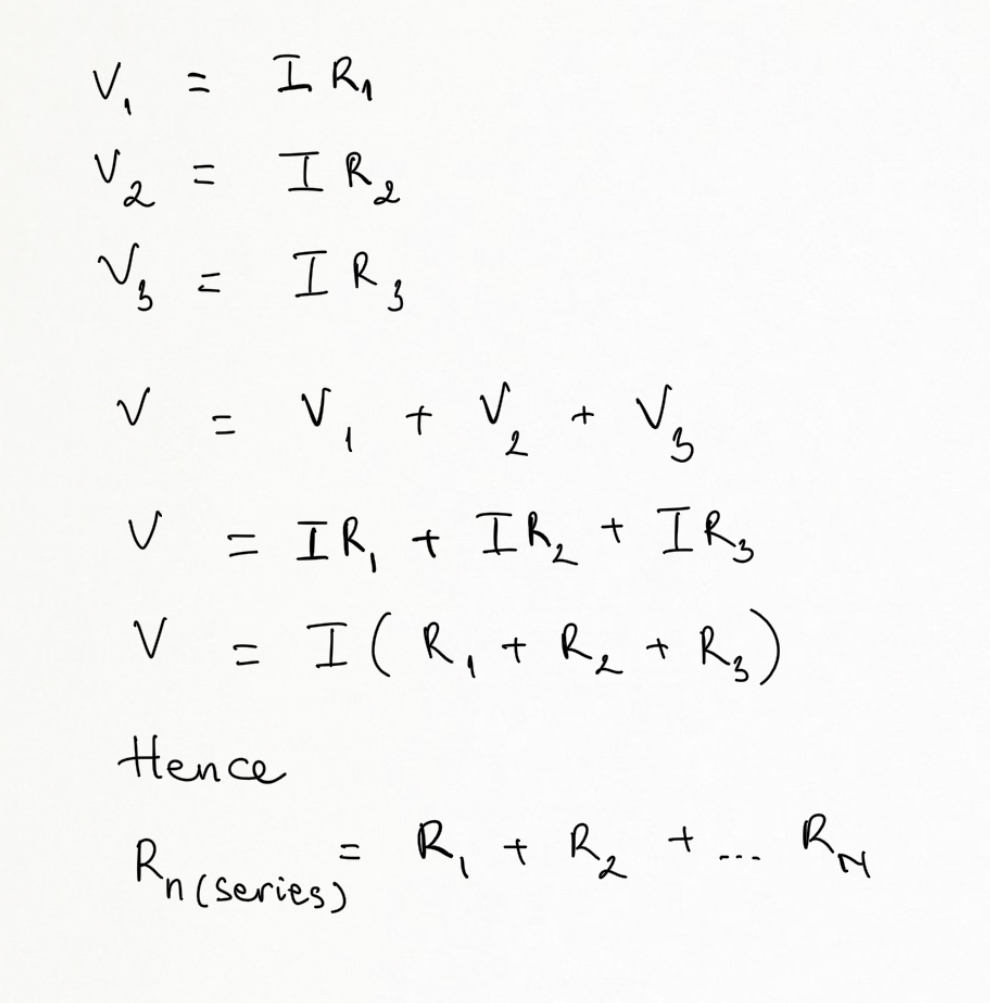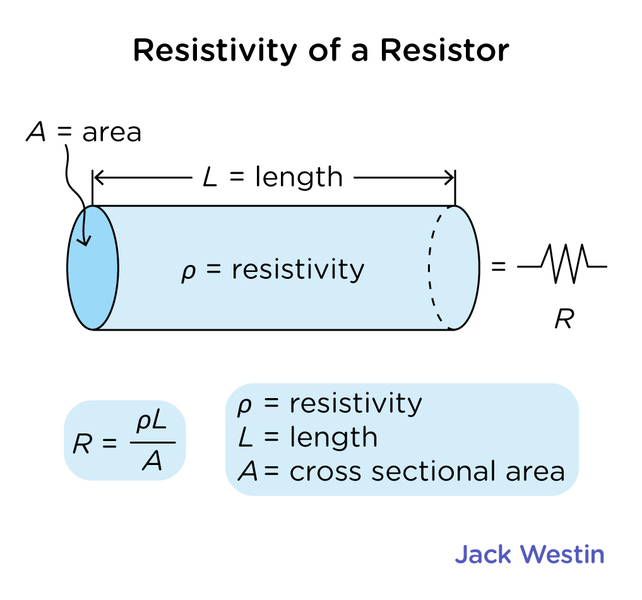Cyber Monday Sale: ALL MCAT Products & Admissions Services

### Resistance

Topic: Circuit Elements

According to Ohm’s law, the voltage drop, V, across a resistor when a current flows through it is calculated by using the equation V=IR, where I is current in amps (A) and R is the resistance in ohms (Ω).

The current that flows through most substances is directly proportional to the voltage V applied to it. The German physicist Georg Simon Ohm (1787–1854) was the first to demonstrate experimentally that the current in a metal wire is directly proportional to the voltage applied. The many substances for which Ohm’s law holds are called ohmic. These include good conductors like copper and aluminum and some poor conductors under certain circumstances. Ohmic materials have a resistance R that is independent of voltage V and current I. An object that has simple resistance is called a resistor, even if its resistance is small.Resistors in Series

Resistors are in series whenever the flow of charge, or the current, must flow through components sequentially.The total resistance in the circuit is equal to the sum of the individual resistances.

Resistors in Parallel

Resistors are in parallel when each resistor is connected directly to the voltage source by connecting wires having negligible resistance. Each resistor thus has the full voltage of the source applied to it.Each resistor in the circuit has the full voltage. According to Ohm’s law, the currents flowing through the individual resistors are I1=VR1The total resistance in a parallel circuit is equal to the sum of the inverse of each individual resistance.Resistivity is the property of a material that quantifies how strongly it resists or conducts electric current. A low resistivity indicates a material that readily allows electric current and vice versa. It is calculated as:

ρ=R•A/L

R is the electrical resistance of a uniform specimen of the material

l is the length of the specimen

A is the cross-sectional area of the specimenPractice Questions

Analyzing electrocardiogram voltage signals

MCAT Official Prep (AAMC)

Physics Online Flashcards Question 1

Physics Question Pack Passage 9 Question 54

Physics Question Pack Passage 9 Question 56

Physics Question Pack Question 117

Section Bank C/P Section Question 15

Section Bank C/P Section Question 17

Sample Test C/P Section Passage 7 Question 35

Practice Exam 1 C/P Section Passage 10 Question 52

Practice Exam 2 C/P Section Question 59

Practice Exam 3 C/P Section Passage 7 Question 39

Key Points

• Voltage drop V across a resistor when a current flows through it is calculated by using the equation V=IR

• The total resistance in the circuit is equal to the sum of the individual resistances.

• The total resistance in a parallel circuit is equal to the sum of the inverse of each individual resistance.

• Resistivity measures how strongly a material resists or conducts electric current.

Key Terms

current: is the amount of charge moving through a cross-section, over a period of time.

voltage: Electrical potential difference, expressed in Volts

resistance: Resistance is a measure of the opposition to current flow in an electrical circuit.

resistivity: the property of a material that quantifies how strongly it resists or conducts electric current

Billing Information